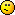<![CDATA[Math Is Fun Forum / Cannot find formula or solution to get answer for feet/inch addition]]> 2022-06-28T09:19:28Z FluxBB https://www.mathisfunforum.com/viewtopic.php?id=26416 <![CDATA[Re: Cannot find formula or solution to get answer for feet/inch addition]]> hi hearpary

Welcome to the forum.

You could do that and get the same answers.

But, looking at the question, it is more an exercise in subtracting fractions, than a question about imperial measures.  Because the conversion multiplier for inches into cm isn't an exact value, the metric answer wouldn't convert back nicely to eighths of an inch.

When we do a number subtraction you may have to use one of these tricks:

71 - 46 = 60 + 11 = 46 so you can do 11 take 6.

Some people use equal addition to do this  71 - 46 = 70 + 11 - (40 +10 + 6)

Either method works.  But, for degree measures you need 60ths not 10ths.  So the second question practises this.

You'd face a similar problem if you had to subtract hours and minutes.

Bob

]]>
https://www.mathisfunforum.com/profile.php?id=67694 2022-06-28T09:19:28Z https://www.mathisfunforum.com/viewtopic.php?pid=425962#p425962
<![CDATA[Re: Cannot find formula or solution to get answer for feet/inch addition]]> Isn't it necessary to convert to the metric system first? I mean from inches to cm.

]]>
https://www.mathisfunforum.com/profile.php?id=240421 2022-06-28T08:45:58Z https://www.mathisfunforum.com/viewtopic.php?pid=425960#p425960
<![CDATA[Re: Cannot find formula or solution to get answer for feet/inch addition]]> Hi Nickm62388;

Here's my way of tackling these (others may have different methods).

Problem #1
The inches & fraction components in the first length are less than their counterparts in the second length, which adds difficulty to subtracting imperial lengths, particularly those with fractions.

To make the subtraction easier, I start with the following three steps:
1. convert the fraction to the Least Common Denominator (LCD); then
2. adjust the value of the fraction component to make it greater than that of the second length; then
3. adjust the value of the inches component to make it greater than that of the second length.

That leaves a simple subtraction exercise.

Btw, the 'take' and 'add' terminology that I used is like 'borrow' and 'pay', now aka 'regrouping', and is how I've always understood that concept.

Also, I've used symbols ' and " instead of abbreviations 'ft' and 'in' respectively...a common notation practice for these imperial length units.Formula: You could convert both lengths to eighths of an inch, deduct the second from the first, and convert the result to feet, inches and eighths:

That last conversion from eighths is a bit tricky...

Problem #2
Same approach as for Problem #1:Formula: You could convert both angles to seconds, deduct the second from the first, and convert the result to degrees, minutes & seconds:

]]>
https://www.mathisfunforum.com/profile.php?id=40741 2021-07-01T05:29:19Z https://www.mathisfunforum.com/viewtopic.php?pid=420079#p420079
<![CDATA[Re: Cannot find formula or solution to get answer for feet/inch addition]]> I appreciate the links, but with the equations I listed. I don’t see how exactly they relate or help answer them with the links.//

]]>
https://www.mathisfunforum.com/profile.php?id=237091 2021-07-01T00:23:39Z https://www.mathisfunforum.com/viewtopic.php?pid=420073#p420073
<![CDATA[Re: Cannot find formula or solution to get answer for feet/inch addition]]> Hi Nickm62388,

Welcome to the forum!

US Standard Lengths.

Degrees (Angles).

Minute (angles).

Second (angles).

]]>
https://www.mathisfunforum.com/profile.php?id=682 2021-07-01T00:04:59Z https://www.mathisfunforum.com/viewtopic.php?pid=420071#p420071
<![CDATA[Cannot find formula or solution to get answer for feet/inch addition]]> I have the answer to these two problems, but cannot figure out how they were got, and cannot find a formula or anything online to get the answer please help

Problem #1

17ft. 7 1/2in.
-  14ft. 9 7/8in.

=??????????

Problem #2

59degrees 37’ 18”
-   40 degrees 43’ 22”

=????? I don’t understand how you subtract something with degrees and feet and inches

Again I have the answers from the answer key but no idea how the answers came to be…